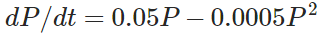# Suppose that a population develops according to the logistic equation

Suppose that a population develops according to the logistic equationWhere t is measured in weeks. What is the carrying capacity? What is the value of k?